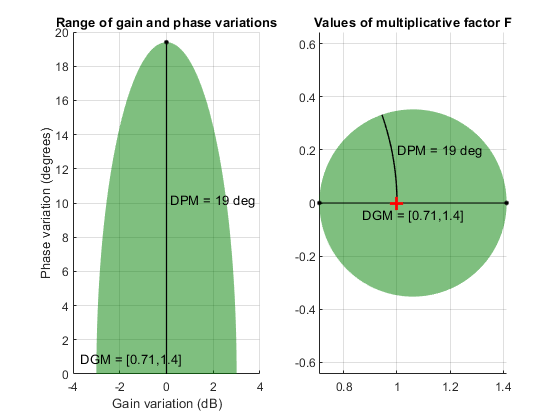## Uncertain Gain and Phase

Use the `umargin` control design block to model gain and phase variations in feedback loops. Modeling gain and phase variations in your uncertain system model lets you verify stability margins during robustness analysis or enforce them during robust controller design.

To add gain and phase uncertainty to a feedback loop, you incorporate `umargin` blocks into an uncertain state-space (`uss`) model of the closed-loop system. `umargin` is a SISO control design block, representing gain and phase variation at a single location in a single feedback loop. To model gain and phase uncertainty in MIMO feedback systems, insert a separate `umargin` object at each location in the system at which you want to introduce gain and phase uncertainty.

### Disk Model of Gain and Phase Uncertainty

`umargin` models gain and phase variations in an individual feedback channel as a frequency-dependent multiplicative factor F(s) multiplying the nominal open-loop response L(s), such that the perturbed response is L(s)F(s). The factor F(s) is parameterized by:

`$F\left(s\right)=\frac{1+\alpha \left[\left(1-\sigma \right)/2\right]\delta \left(s\right)}{1-\alpha \left[\left(1+\sigma \right)/2\right]\delta \left(s\right)}.$`

In this model,

• δ(s) is a gain-bounded dynamic uncertainty, normalized so that it always varies within the unit disk (||δ|| < 1).

• ɑ sets the amount of gain and phase variation modeled by F. For fixed σ, the parameter ɑ controls the size of the disk. For ɑ = 0, the multiplicative factor is 1, corresponding to the nominal L.

• σ, called the skew, biases the modeled uncertainty toward gain increase or gain decrease.

The factor F takes values in a disk centered on the real axis and containing the nominal value F = 1. The disk is characterized by its intercept `DGM = [gmin,gmax]` with the real axis. `gmin` < 1 and `gmin` > 1 are the minimum and maximum relative changes in gain modeled by F, at nominal phase. The phase uncertainty modeled by F is the range `DPM = [pmin,pmax]` of phase values at the nominal gain (|F| = 1). For instance, in the following plot, the right side shows the disk F that intersects the real axis in the interval [0.71,1.4]. The left side shows that this disk models a gain variation of ±3 dB and a phase variation of ±19°.

```F = umargin('F',1.4125) plot(F)```When you create a `umargin` block, you specify the amount of uncertainty by specifying `DGM`. Use `getDGM` to translate specific amounts of gain and phase variations in to a suitable `DGM` range that captures these variations. For more information about the uncertainty model used by `umargin`, see Stability Analysis Using Disk Margins.

You can visualize the ranges of gain and phase uncertainty represented by a `umargin` object using ```plot (umargin)```.

For examples of creating `umargin` objects and incorporating them into uncertain models, see:

### Using Gain and Phase Uncertainty

When you have a `uss` model containing `umargin` control design blocks, you can perform robustness and worst-case analysis to examine how gain and phase variation affects the response of the system. For instance, use `robstab` and `robgain` to analyze the robust stability and robust performance of a system with gain and phase uncertainty. Use `wcgain` and `wcsigmaplot` to examine the worst-case responses of the system. For some examples, see:

Requiring robust stability for a closed-loop system with `umargin` gain and phase uncertainty is equivalent to enforcing a disk-based gain margin `[gmin,gmax]` and corresponding phase margin. Therefore, you can use `umargin` blocks to enforce suitable disk margins when designing robust controllers with `musyn`. For examples, see:

The requirement that a closed-loop system is robust against a particular amount of gain and phase uncertainty is equivalent to saying that the system has that amount of gain and phase margin. You can therefore use a `umargin` block to check the disk-based stability margins of a system that also requires robustness against other types of uncertainty. For an example, see: